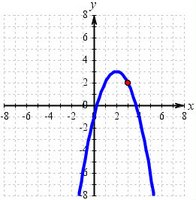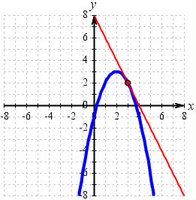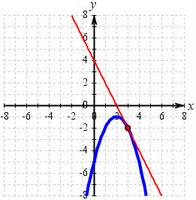## Saturday, December 16, 2006

### The terrible math problemTerribly easy? or confusing?

The bane of a math teacher's existence is the egregiously incorrect solution that nevertheless produces a correct answer. Students hate it when they don't get full credit for having the “right” answer, although that answer is no more than an extremely lucky happenstance.

A running tally of such “Lucky Larry” solutions is maintained by the American Mathematical Association of Two-Year Colleges. The examples come from all mathematical fields, but algebra is particularly ripe territory for the efforts of Lucky Larry, with bogus cancellation a classic case. Whatever the subject, the key element is coincidence.

Since I disdain multiple-choice exams in my math classes, no one is going to get credit on a problem by means of a Lucky Larry solution. However, I have to read my students' work carefully enough to make certain that I catch any illogical leaps in their steps. I make my task all the more difficult when I set up a perfect Lucky Larry situation, as I did in a recent calculus exam. Although I had carefully worked out the solution key in advance, I missed the trap I had set for myself (and for my students!):
(a) Find the slope of the graph of f(x) = −x2 + 4x − 1 at the point (3, 2).

(b) Find the slope-intercept equation of the tangent line at (3, 2) and sketch it on the given graph.What could be simpler or more straightforward? The derivative is f′(x) = −2x + 4. If we evaluate the derivative at x = 3, we obtain f′(3) = −2(3) + 4 = −6 + 4 = −2. The slope of the tangent line at (3, 2) is −2.

But wait a minute. A few of my students took the derivative, looked at −2x + 4, observed that the slope is given by the coefficient of the x term, and cheerfully wrote down m = −2. Correct answer. Wrong reason!Nevertheless, it could have been even worse. In the second part of the problem I asked my students to find the slope-intercept equation of the tangent line through (3, 2). The result is supposed to be y = −2x + 8. When added to the graph, it makes a very nice tangent line indeed, confirming the results of our calculation. No problem.

However, what if I had chosen f(x) = −x2 + 4x − 5 as my quadratic function and (3, −2) as my tangency point? The derivative would be the same as that of the function I actually chose for my exam, f′(x) = −2x + 4, but the equation of its tangent line would be y = −2x + 4 in this case. That's right: The derivative function would equal the tangent's linear function. That's a big fat Lucky Larry just waiting to happen.I can't resist sharing this one with my colleagues, of course, despite the imprudence of such an action. Once it's embedded in our brain cells, will it be waiting to manifest itself during our next exam-writing session? Or might we accidentally use it as an example during a lecture and then have to spend the rest of the semester “unteaching” our students a wonderfully invalid shortcut?

Beware!Anonymous said...

My own favourite is the volume of a paraboloid of rotation, which is exactly one half that of the inscribed cylinder. So you get the correct answer if you mess up the limits of integration and integrate from z=0 to z=r² instead of from z=r² to z=h.

Jokermage said...

I'm just glad I remember enough high school math to get the right answer for part b. I'm also glad I went to a high school that taught introductory calculus to some of its students.Anonymous said...

My theory of the bane of shortcuts is that it is never filed in the brain... shortcuts bash around in the brain without a context and come out almost at random.

You have just given hope to this math teacher... who reads each problem on finals that get filed away without most students seeing them. :-)